## Tamilnadu Samacheer Kalvi 10th Maths Solutions Chapter 8 Statistics and Probability Ex 8.1

10th Maths Exercise 8.1 Samacheer Kalvi Question 1.
Find the range and coefficient of range of the following data.
(i) 63, 89, 98, 125, 79, 108, 117, 68
(ii) 43.5, 13.6, 18.9, 38.4, 61.4, 29.8
Solution:
Range R = L – S.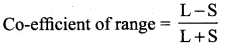L – Largest value,
S – Smallest value.

(i) 63, 89, 98, 125, 79, 108, 117, 68.
Here L = 125
S = 63
∴ R = L – S = 125 – 63 = 62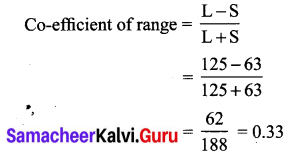Exercise 8.1 Class 10 Samacheer Question 2.
If the range and the smallest value of a set of data are 36.8 and 13.4 respectively, then find the largest value.
Range = 36.8
Smallest value (S) = 13.4
Range = L – S
36.8 = L – 13.4
L = 36.8 + 13.4 = 50.2
Largest value = 50.2

Ex 8.1 Class 10 Samacheer Question 3.
Calculate the range of the following data.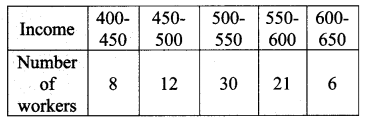Solution:
Here the largest value = 650
The smallest value =400
∴ Range = L – S = 650 – 400
= 250

10th new syllabus maths exercise 8.1 Question 4.
A teacher asked the students to complete 60 pages of a record notebook. Eight students have completed only 32, 35, 37, 30, 33, 36, 35 and 37 pages. Find the standard deviation of the pages yet to be completed by them.
Solution: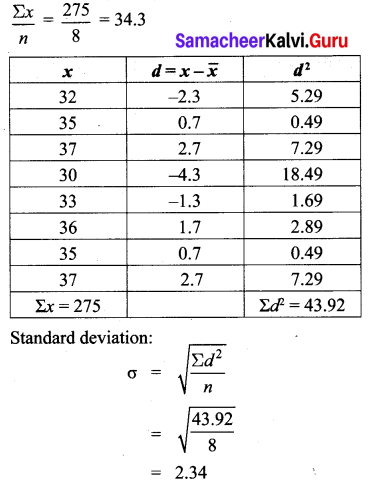10th Maths Statistics And Probability Question 5.
Find the variance and standard deviation of the wages of 9 workers given below:
₹ 310, ₹ 290, ₹ 320, ₹ 280, ₹ 300, ₹ 290, ₹ 320, ₹ 310, ₹ 280.
Solution: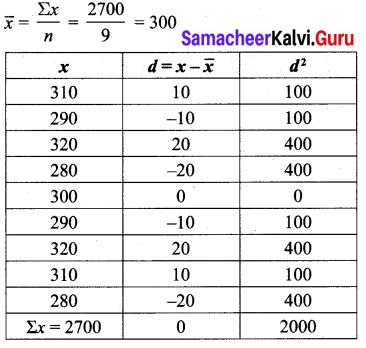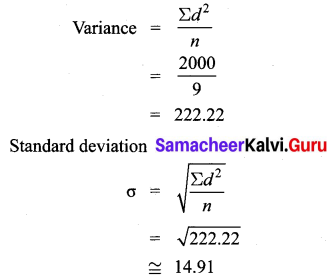10th Maths Exercise 8.1 Question 6.
A wall clock strikes the bell once at 1 o’clock, 2 times at 2 o’clock, 3 times at 3 o’clock and so on. How many times will it strike in a particular day? Find the standard deviation of the number of strikes the bell make a day.
Solution: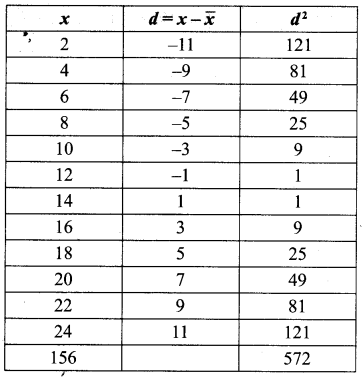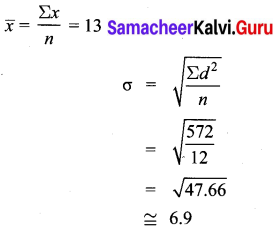10th Maths Ex 8.1 Question 7.
Find the standard deviation of the first 21 natural numbers.
Solution: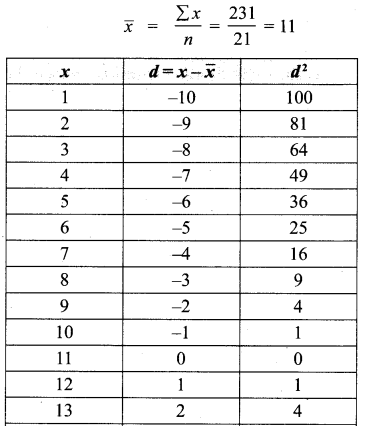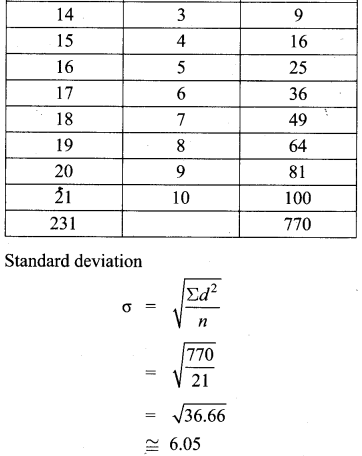10th Maths Exercise 8.1 In Tamil Question 8.
If the standard deviation of a data is 4.5 and if each value of the data is decreased by 5, then find the new standard deviation.
The standard deviation of the data = 4.5
Each data is decreased by 5
The new standard deviation = 4.5

10th Maths 8.1 Question 9.
If the standard deviation of a data is 3.6 and each value of the data is divided by 3, then find the new variance and new standard deviation.
Solution:
If the standard deviation of a data is 3.6, and each of the data is divided by 3 then the new standard deviation is also divided by 3.
∴ The new standard deviation = $$\frac{3.6}{3}$$
= 1.2
The new variance = (standard deviation)2
$$=\sigma^{2}=1.2^{2}=1.44$$

10th Maths Chapter 8 Exercise 8.1 Question 10.
The rainfall recorded in various places of five districts in a week are given below.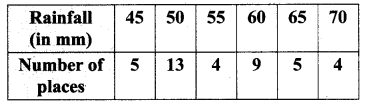Find its standard deviation.
Solution: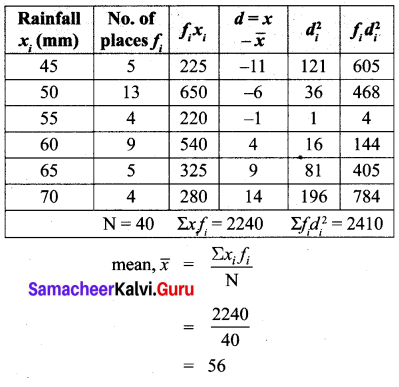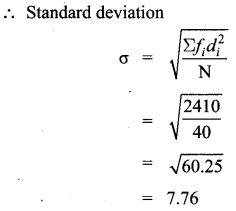10th Maths 8.1 Tamil Question 11.
In a study about viral fever, the number of people affected in a town were noted as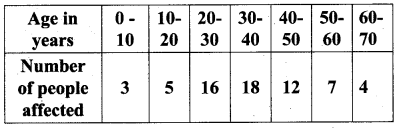Find its standard deviation.
Solution:
Let the assumed mean A = 35, C = 10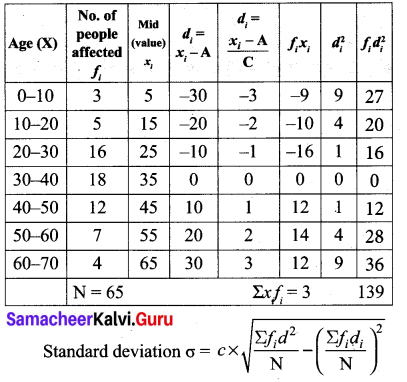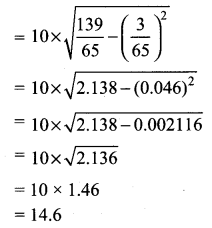10th Maths 8th Chapter Question 12.
The measurements of the diameters (in cms) of the plates prepared in a factory are given below. Find its standard deviation.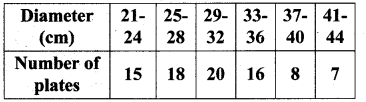Solution:
Assumed mean A = 30.5, C = 4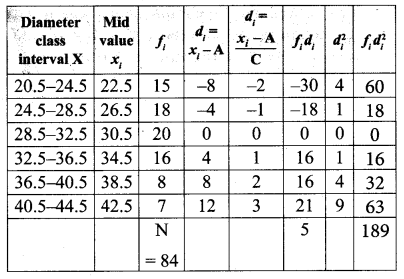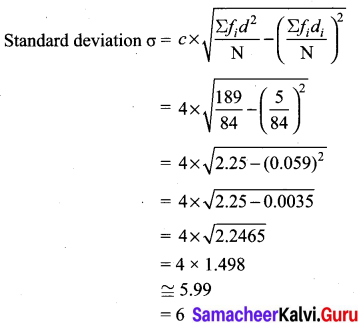12th Maths Exercise 8.1 Samacheer Kalvi Question 13.
The time taken by 50 students to complete a 100 meter race are given below. Find its standard deviation.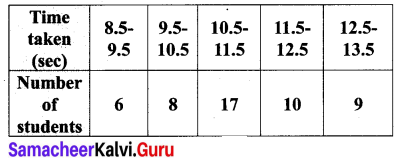Solution:
Assumed mean A = 11, C = 1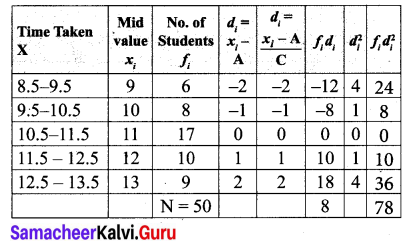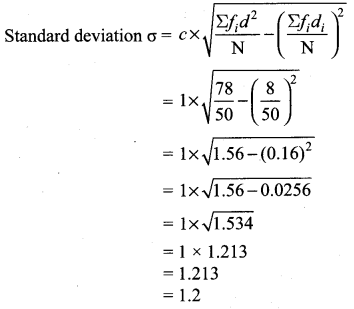Samacheer Kalvi Guru 10th Maths Question 14.
For a group of 100 candidates the mean and standard deviation of their marks were found to be 60 and 15 respectively. Later on it was found that the scores 45 and 72 were wrongly entered as 40 and 27. Find the correct mean and standard deviation.
Solution: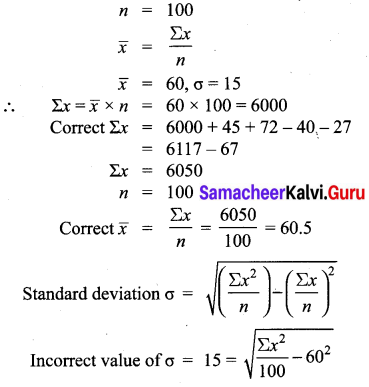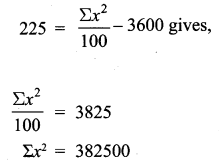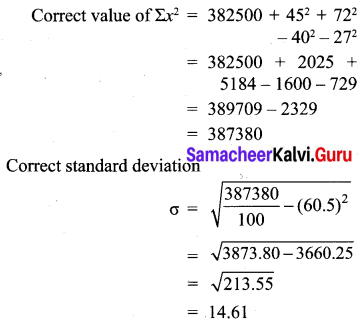Samacheer Kalvi.Guru 10th Question 15.
The mean and variance of seven observations are 8 and 16 respectively. If five of these are 2, 4, 10, 12 and 14, then find the remaining two observations.
Solution: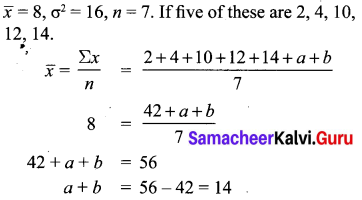>
The given 5 number are 2, 4, a, b, 10, 12, 14.
a, b are 6 and 8.